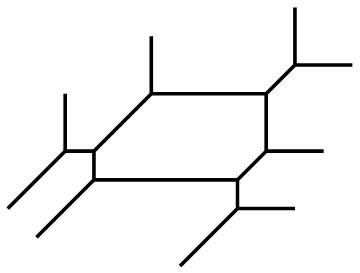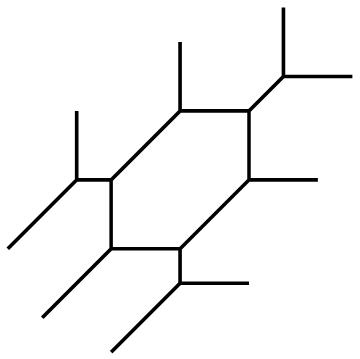Elliptic Curves in Honeycomb Form

### Supplementary materials for Elliptic Curves in Honeycomb Form, M. Chan and B. SturmfelsFormula for the j-invariant of a ternary cubic The j-invariant of a ternary cubic c111*x*y*z + c210*x^2*y + c201*x^2*z + c120*x*y^2 + c102*x*z^2 +c021*y^2*z + c012*y*z^2 + c300*x^3 + c030*y^3 + c003*z^3 can be expressed as a rational function in its ten coefficients as in the formula above. We computed this rational function by writing j=S^3/(T^2-64S^3), where S and T are the degree 4 and degree 6 invariants of a ternary cubic, respectively, explicit formulae for which are given in Algorithms in Invariant Theory, B. Sturmfels, Proposition 4.4.7 and Example 4.5.3. Maple computation and Macaulay2 computation for Lemma 3 We compute the ideal describing the condition that a linear form divides a ternary cubic. The maple code is used to produce the input to Macaulay2. Specializing this ideal to the case that the cubic is in the Hesse pencil of a given cubic f gives a Grobner basis whose triangular form shows that the inflection points lie in a solvable extension. The full output is here. Mathematica computation of the inflection points of a general smooth cubic This Mathematica code carries out the computation described in Section 2 to compute an explicit formula, in radicals, for the nine inflection points of a general smooth cubic. The full formula, which occupies 33,013 KB, can be found here. Note that the invariants S and T are left unexpanded in terms of the ten coefficients; otherwise, the formula would be many gigabytes long. Computation of a 3x3 matrix taking a given cubic to honeycomb form (forthcoming) Mathematica algorithm to compute honeycomb embeddings We illustrate our Theorem 7 by producing, for a given j-invariant and a given choice of nine nonzero field elements p1,...,p9 satisfying the conditions of Section 3, a parametrization by theta functions of the associated honeycomb embedding, and the coefficients cijk which give the implicit representation of our honeycomb elliptic curve. Our code is demonstrated on a sample choice of nine points given in Section 4.